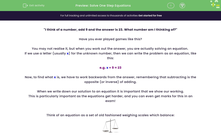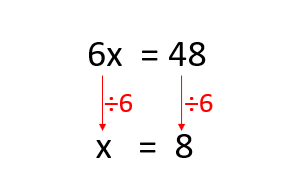# Solve One Step Equations

In this worksheet, students will learn how to solve one-step equations by finding the value of one unknown element represented by a letter.Key stage:  KS 4

Year:  GCSE

GCSE Subjects:   Maths

GCSE Boards:   Pearson Edexcel, OCR, Eduqas, AQA,

Curriculum topic:   Algebra

Curriculum subtopic:   Solving Equations and Inequalities Algebraic Expressions

Difficulty level:#### Worksheet Overview

"I think of a number, add 9 and the answer is 23. What number am I thinking of?"

Have you ever played games like this?

You may not realise it, but when you work out the answer, you are actually solving an equation.

If we use a letter (usually x) for the unknown number, then we can write the problem as an equation, like this:

e.g. x + 9 = 23

Now, to find what x is, we have to work backwards from the answer, remembering that subtracting is the opposite (or inverse) of adding.

When we write down our solution to an equation it is important that we show our working.

This is particularly important as the equations get harder, and you can even get marks for this in an exam!

Think of an equation as a set of old fashioned weighing scales which balance:If you add or take something from one side of the scales, it will upset the balance, unless you so the same thing to the other side.

When we solve an equation we are trying to get the x by itself on the left-hand side.

To do this with our example above, we must remove the + 9 by doing the inverse which is - 9.

However, this will upset the balance unless we - 9 from the right-hand side as well.

We set these steps out like this:So the solution to the equation is: x = 14

Let's try another equation now:

e.g. 6x = 48

What does 6x mean?  It means 6 multiplied by x.

Remember the scales, what we do to one side of the equation, we must do to the other.

Since the inverse of multiply is divide we set out our working as follows:Remember, x is not always used as the letter to represent the unknown element, any letter could be used but the principle is still the same!

Let's give this a go now!

In this activity, we will solve equations by finding the value of one unknown element represented by a letter.

### What is EdPlace?

We're your National Curriculum aligned online education content provider helping each child succeed in English, maths and science from year 1 to GCSE. With an EdPlace account you’ll be able to track and measure progress, helping each child achieve their best. We build confidence and attainment by personalising each child’s learning at a level that suits them.

Get started